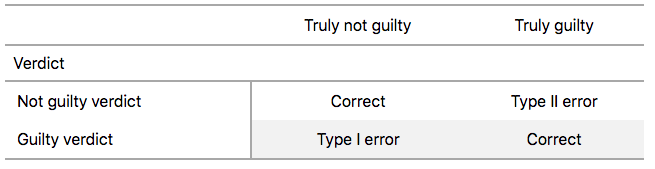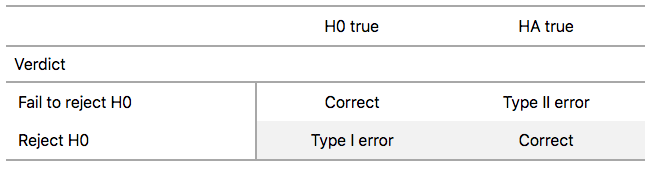## 9.4 Interpreting hypothesis tests

Interpreting the results of hypothesis tests is one of the more challenging aspects of this method for statistical inference. In this section, we’ll focus on ways to help with deciphering the process and address some common misconceptions.

### 9.4.1 Two possible outcomes

In Section 9.2, we mentioned that given a pre-specified significance level $$\alpha$$ there are two possible outcomes of a hypothesis test:

• If the $$p$$-value is less than $$\alpha$$, then we reject the null hypothesis $$H_0$$ in favor of $$H_A$$.
• If the $$p$$-value is greater than or equal to $$\alpha$$, we fail to reject the null hypothesis $$H_0$$.

Unfortunately, the latter result is often misinterpreted as “accepting the null hypothesis $$H_0$$.” While at first glance it may seem that the statements “failing to reject $$H_0$$” and “accepting $$H_0$$” are equivalent, there actually is a subtle difference. Saying that we “accept the null hypothesis $$H_0$$” is equivalent to stating that “we think the null hypothesis $$H_0$$ is true.” However, saying that we “fail to reject the null hypothesis $$H_0$$” is saying something else: “While $$H_0$$ might still be false, we don’t have enough evidence to say so.” In other words, there is an absence of enough proof. However, the absence of proof is not proof of absence.

To further shed light on this distinction, let’s use the United States criminal justice system as an analogy. A criminal trial in the United States is a similar situation to hypothesis tests whereby a choice between two contradictory claims must be made about a defendant who is on trial:

1. The defendant is truly either “innocent” or “guilty.”
2. The defendant is presumed “innocent until proven guilty.”
3. The defendant is found guilty only if there is strong evidence that the defendant is guilty. The phrase “beyond a reasonable doubt” is often used as a guideline for determining a cutoff for when enough evidence exists to find the defendant guilty.
4. The defendant is found to be either “not guilty” or “guilty” in the ultimate verdict.

In other words, not guilty verdicts are not suggesting the defendant is innocent, but instead that “while the defendant may still actually be guilty, there wasn’t enough evidence to prove this fact.” Now let’s make the connection with hypothesis tests:

1. Either the null hypothesis $$H_0$$ or the alternative hypothesis $$H_A$$ is true.
2. Hypothesis tests are conducted assuming the null hypothesis $$H_0$$ is true.
3. We reject the null hypothesis $$H_0$$ in favor of $$H_A$$ only if the evidence found in the sample suggests that $$H_A$$ is true. The significance level $$\alpha$$ is used as a guideline to set the threshold on just how strong of evidence we require.
4. We ultimately decide to either “fail to reject $$H_0$$” or “reject $$H_0$$.”

So while gut instinct may suggest “failing to reject $$H_0$$” and “accepting $$H_0$$” are equivalent statements, they are not. “Accepting $$H_0$$” is equivalent to finding a defendant innocent. However, courts do not find defendants “innocent,” but rather they find them “not guilty.” Putting things differently, defense attorneys do not need to prove that their clients are innocent, rather they only need to prove that clients are not “guilty beyond a reasonable doubt”.

So going back to our résumés activity in Section 9.3, recall that our hypothesis test was $$H_0: p_{m} - p_{f} = 0$$ versus $$H_A: p_{m} - p_{f} > 0$$ and that we used a pre-specified significance level of $$\alpha$$ = 0.05. We found a $$p$$-value of 0.027. Since the $$p$$-value was smaller than $$\alpha$$ = 0.05, we rejected $$H_0$$. In other words, we found needed levels of evidence in this particular sample to say that $$H_0$$ is false at the $$\alpha$$ = 0.05 significance level. We also state this conclusion using non-statistical language: we found enough evidence in this data to suggest that there was gender discrimination at play.

### 9.4.2 Types of errors

Unfortunately, there is some chance a jury or a judge can make an incorrect decision in a criminal trial by reaching the wrong verdict. For example, finding a truly innocent defendant “guilty”. Or on the other hand, finding a truly guilty defendant “not guilty.” This can often stem from the fact that prosecutors don’t have access to all the relevant evidence, but instead are limited to whatever evidence the police can find.

The same holds for hypothesis tests. We can make incorrect decisions about a population parameter because we only have a sample of data from the population and thus sampling variation can lead us to incorrect conclusions.

There are two possible erroneous conclusions in a criminal trial: either (1) a truly innocent person is found guilty or (2) a truly guilty person is found not guilty. Similarly, there are two possible errors in a hypothesis test: either (1) rejecting $$H_0$$ when in fact $$H_0$$ is true, called a Type I error or (2) failing to reject $$H_0$$ when in fact $$H_0$$ is false, called a Type II error. Another term used for “Type I error” is “false positive,” while another term for “Type II error” is “false negative.”

This risk of error is the price researchers pay for basing inference on a sample instead of performing a census on the entire population. But as we’ve seen in our numerous examples and activities so far, censuses are often very expensive and other times impossible, and thus researchers have no choice but to use a sample. Thus in any hypothesis test based on a sample, we have no choice but to tolerate some chance that a Type I error will be made and some chance that a Type II error will occur.

To help understand the concepts of Type I error and Type II errors, we apply these terms to our criminal justice analogy in Figure 9.15.FIGURE 9.15: Type I and Type II errors in criminal trials.

Thus a Type I error corresponds to incorrectly putting a truly innocent person in jail, whereas a Type II error corresponds to letting a truly guilty person go free. Let’s show the corresponding table in Figure 9.16 for hypothesis tests.FIGURE 9.16: Type I and Type II errors in hypothesis tests.

### 9.4.3 How do we choose alpha?

If we are using a sample to make inferences about a population, we run the risk of making errors. For confidence intervals, a corresponding “error” would be constructing a confidence interval that does not contain the true value of the population parameter. For hypothesis tests, this would be making either a Type I or Type II error. Obviously, we want to minimize the probability of either error; we want a small probability of making an incorrect conclusion:

• The probability of a Type I Error occurring is denoted by $$\alpha$$. The value of $$\alpha$$ is called the significance level of the hypothesis test, which we defined in Section 9.2.
• The probability of a Type II Error is denoted by $$\beta$$. The value of $$1-\beta$$ is known as the power of the hypothesis test.

In other words, $$\alpha$$ corresponds to the probability of incorrectly rejecting $$H_0$$ when in fact $$H_0$$ is true. On the other hand, $$\beta$$ corresponds to the probability of incorrectly failing to reject $$H_0$$ when in fact $$H_0$$ is false.

Ideally, we want $$\alpha = 0$$ and $$\beta = 0$$, meaning that the chance of making either error is 0. However, this can never be the case in any situation where we are sampling for inference. There will always be the possibility of making either error when we use sample data. Furthermore, these two error probabilities are inversely related. As the probability of a Type I error goes down, the probability of a Type II error goes up.

What is typically done in practice is to fix the probability of a Type I error by pre-specifying a significance level $$\alpha$$ and then try to minimize $$\beta$$. In other words, we will tolerate a certain fraction of incorrect rejections of the null hypothesis $$H_0$$, and then try to minimize the fraction of incorrect non-rejections of $$H_0$$.

So for example if we used $$\alpha$$ = 0.01, we would be using a hypothesis testing procedure that in the long run would incorrectly reject the null hypothesis $$H_0$$ one percent of the time. This is analogous to setting the confidence level of a confidence interval.

So what value should you use for $$\alpha$$? Different fields have different conventions, but some commonly used values include 0.10, 0.05, 0.01, and 0.001. However, it is important to keep in mind that if you use a relatively small value of $$\alpha$$, then all things being equal, $$p$$-values will have a harder time being less than $$\alpha$$. Thus we would reject the null hypothesis less often. In other words, we would reject the null hypothesis $$H_0$$ only if we have very strong evidence to do so. This is known as a “conservative” test.

On the other hand, if we used a relatively large value of $$\alpha$$, then all things being equal, $$p$$-values will have an easier time being less than $$\alpha$$. Thus we would reject the null hypothesis more often. In other words, we would reject the null hypothesis $$H_0$$ even if we only have mild evidence to do so. This is known as a “liberal” test.

Learning check

(LC9.5) What is wrong about saying, “The defendant is innocent.” based on the US system of criminal trials?

(LC9.6) What is the purpose of hypothesis testing?

(LC9.7) What are some flaws with hypothesis testing? How could we alleviate them?

(LC9.8) Consider two $$\alpha$$ significance levels of 0.1 and 0.01. Of the two, which would lead to a more liberal hypothesis testing procedure? In other words, one that will, all things being equal, lead to more rejections of the null hypothesis $$H_0$$.